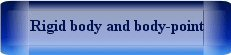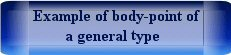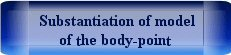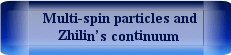Elena A. Ivanova St. Petersburg State Polytechnical UniversityNon-classical particles and continua consisting of these particles

The opinion that classical mechanics is inapplicable in microcosm is well known. However, the question arises: What kind of mechanics is implied when it is stated that classical mechanics is not applicable in microcosm? The answer is quite obvious. We are talking about Newtonian mechanics (mechanics of mass points) which is usually set out in courses on physics. It makes little sense to dispute the fact that models of Newtonian mechanics do not allow us to describe the processes occurring in microcosm. Indeed, many attempts to construct an atomic model starting with the concept of a discrete system of mass points which interacts by central forces have been unsuccessful. Attempts to construct models of the electromagnetic field which are based on the concept of an elastic continuum consisting of mass points were also unsuccessful. However, the above-mentioned failures prove only scantiness of Newtonian mechanics and the inadequacy of its models to those processes and phenomena which are observed in microcosm. They do not prove the impossibility of describing phenomena in microcosm on the basis of the fundamental laws of classical mechanics. Newtonian mechanics in a form that is presented in the modern textbooks on physics was created more than two hundred years ago. For two hundred years classical mechanics has been developed significantly. The fundamental laws of mechanics that are considerably more general than Newton's second law have been formulated. In the framework of classical mechanics models of particles that are more complex than the models of mass point and rigid body have been suggested. Based on these models of particles the models of media possessing both mechanical and non-mechanical properties have been constructed.

Model of body-point

The fundamental laws that are formulated for arbitrary body are the basis of classical mechanics. In fact the fundamental laws are the equations of balance between the rate of change of quantities characterizing the state of body and quantities that characterize the interaction of body with outward things. The method of classical mechanics is to choose an adequate model of the body, to formulate the constitutive equations for quantities characterizing the interaction of body with its surroundings, and then to apply the fundamental laws. If classical mechanics is understood as a method of studying physical processes, it has within itself no restrictions to its field of application. Specific models of classical mechanics are of limited applicability. Therefore, the prospects for the development of classical mechanics and its extension to such areas that were previously considered to be not subservient to classical mechanics are connected with the creation and study of more complicated base models. The model of mass point is the simplest basic model in classical mechanics. This is followed by an infinitesimal rigid body, i.e. the particle that occupies zero volume in space, possesses not only translational but also rotational degrees of freedom, and is the exact analogue of the macroscopic rigid body by the dynamic properties. The next in complexity basic object is the body-point of a general type. The body-point is a particle that occupies zero volume in space, possesses translational and rotational degrees of freedom, and is characterized by the mass and two tensors of inertia, one of which is symmetric, and the second can be arbitrary. The formal definition of body-point is the assignment of its kinetic energy as a quadratic form of translational and angular velocities. The coefficients of this quadratic form are the inertia tensors of body-point. A mass point and an infinitesimal rigid body are special cases of the body-point. At the same time, the definition of body-point assumes the existence of body-points which have additional inertial characteristics compared with the infinitesimal rigid bodies. Such body-points are called body-points of a general type or non-classical particles. The latter name is due to the fact that the body-points of a general type are fundamentally different in their dynamic properties from the infinitesimal rigid bodies. In particular, the free motion of the body-point of a general type is not the motion in a straight line. The presence of additional inertial characteristics of the body-point of a general type should be treated as taking into account (in the integral sense) the internal dynamics of a complex particle possessing internal degrees of freedom. We can give an example of the mechanical system with a complex internal structure, the approximate description of which motion are reduced to the equations of motion of the body-point different form the infinitesimal rigid body.Models of particles used in continuum mechanics

In the case of the description of dynamics of a system consisting of a large number of particles, the difference between mechanics of discrete media and continuum mechanics is purely mathematical. In the case of mechanics of discrete media the motion of system of particles is described by a large number of ordinary differential equations. In the case of continuum mechanics the main variables are the characteristics of a representative volume of medium, which are actually the values averaged over the particles that are in the representative volume. With this approach, the description of motion of the medium is reduced to a system of partial differential equations. It is important to note that the dynamic properties of representative volume of continuous media have no qualitatively difference from the dynamic properties of particles that are within this representative volume. All particles discussed above can be used as the base models for the construction of various continuum theories. For example, the model of mass point is the basis of momentless continuum theories: classical three-dimensional theory of elasticity, the theory of membranes and the theory of filaments. The model of infinitesimal rigid body is the basis of moment continuum theories: the theory of beams and rods, the theory of plates and shells, three-dimensional theories based on the Cosserat continua. The model of infinitesimal rigid body, the model of body-point of a general type, as well as models of multi-spin particles (particles with internal rotational degrees of freedom) can be used to describe the various "non-mechanical processes" on the basis of the fundamental laws of classical mechanics. Certainly, this continuous media should be understood in a wide sense. We mean by them not only ponderable matter, but also the physical fields, i.e. everything that is described by differential equations in partial derivatives.One-rotor gyrostat continuum as a model of thermoviscoelastic medium

The one-rotor gyrostat is a complex object which consists of the carrier body and the rotor (see Figure). The rotor can rotate independently of rotation of the carrier body, but it can not translate relative to the carrier body. Further we discuss the one-rotor gyrostats of a special type. The carrier bodies of the gyrostats are the infinitesimal rigid bodies but rotors of the gyrostats represent body-points whose tensors of inertia are the spherical parts of tensors. Let us consider the material medium (see Figure) consisting of the aforesaid one-rotor gyrostats. The particles of continuum under consideration possess the internal degrees of freedom. The main features of the method of modelling of continuum with internal rotational degrees of freedom are following. In order to describe the motion of this continuum it is not sufficient to formulate the balance equations of the momentum and the angular momentum for the control volume of the continuum. It is necessary to add these equations to the balance equation of the angular momentum for the rotors in control volume of the continuum. Moreover, the energy balance equation contains the additional terms. Some of them can be considered as the rate of energy supply.One-rotor gyrostat and a representative volume in continuum consisting of one-rotor-gyrostats

Based on the mechanical model of a one-rotor gyrostat continuum a new approach to derivation of the theory of thermoviscoelasticity is suggested. It is proved that this mechanical model can be used for description of thermal and dissipative phenomena. Interpretation of the temperature, entropy and other thermodynamic quantities given in accordance with the suggested model is no more than the mechanical analogy. However, use of these mechanical counterparts allows one to obtain the well-known equations describing the thermal and diffusion processes within the framework of the model. The mathematical description of the suggested mechanical model includes as special cases not only the classical formulation of coupled problem of thermoelasticity but also the formulation of the coupled problem of thermoelasticity with the hyperbolic type heat conduction equation. In the context of the suggested mechanical model, an original interpretation of the volume (acoustic) viscosity and the shear viscosity is suggested. The suggested description of the internal damping are based on the results of solving the model problems of interaction of a body-point with the surrounding medium.The derivation of theory of thermoviscoelasticity on the base of the mechanical model of one-rotor gyrostat continuum can be found in the papers:

Conclusion

It is the presence of additional rotational degrees of freedom and, accordingly, additional inertia and elastic characteristics which can be interpreted as the non-mechanical constants that distinguish the models based on the complex particles among other continual models. If the mathematical description of some continual model can be reduced to the known physical equations (for example, the heat conduction equation, the electrodynamics equations, etc.) then the continual model can be considered as the mechanical model of the corresponding physical process.